# Enolates – Organic Chemistry – list of solved problems

Go to the page with the solutions to the problems.

## Enolates – list of problems

### Problem 1)

Complete the following reactions: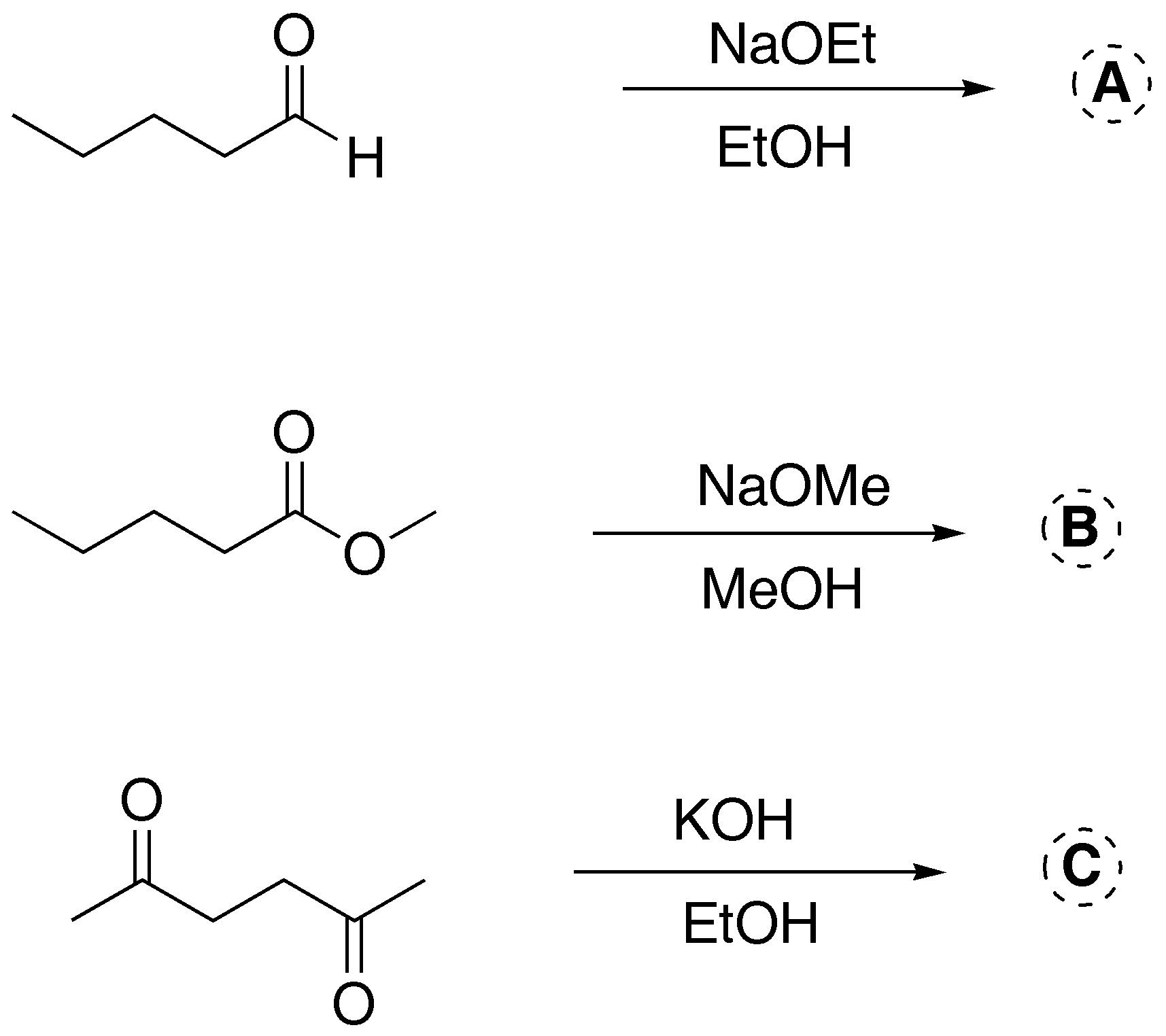### Problem 2)

What reactants are necessary to perform the following transformations?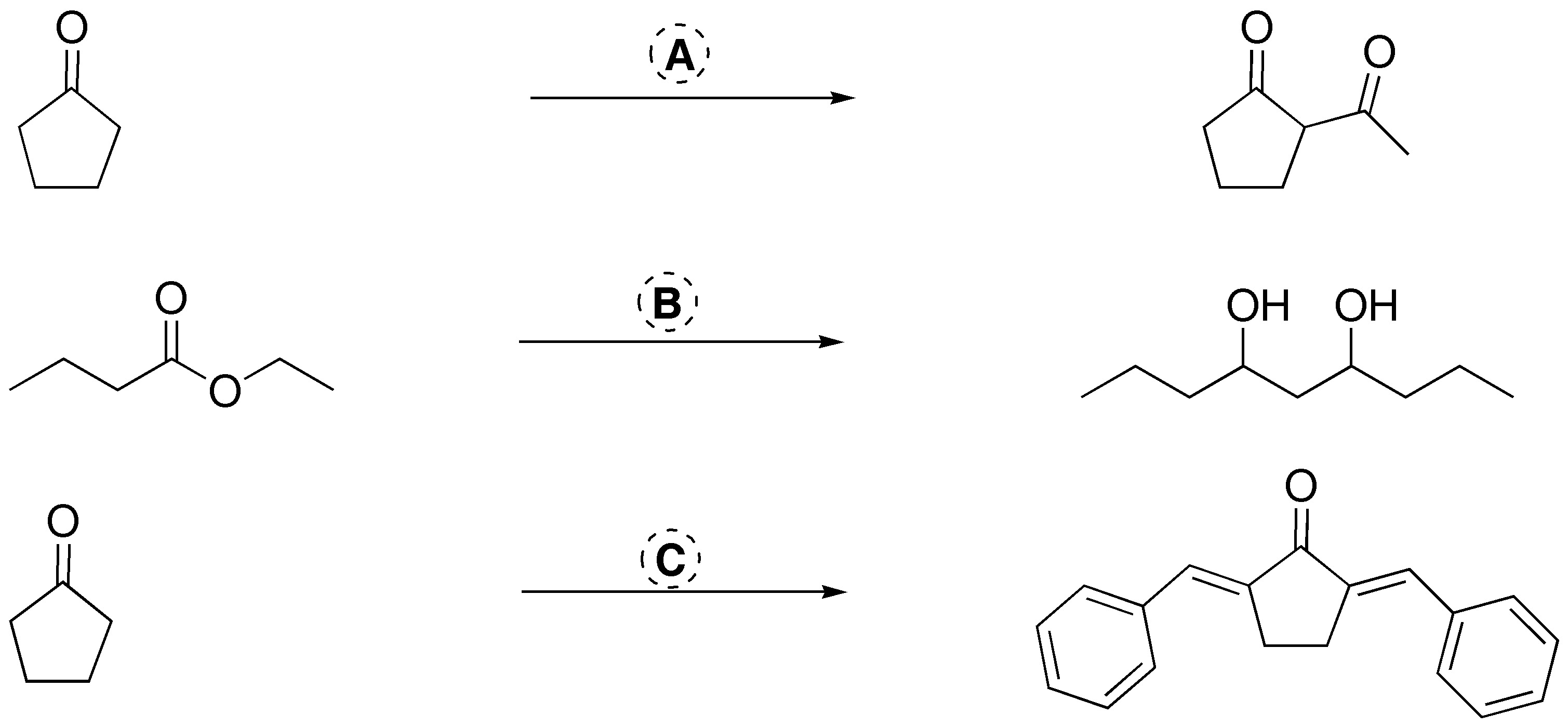### Problem 3)

Complete the following reactions: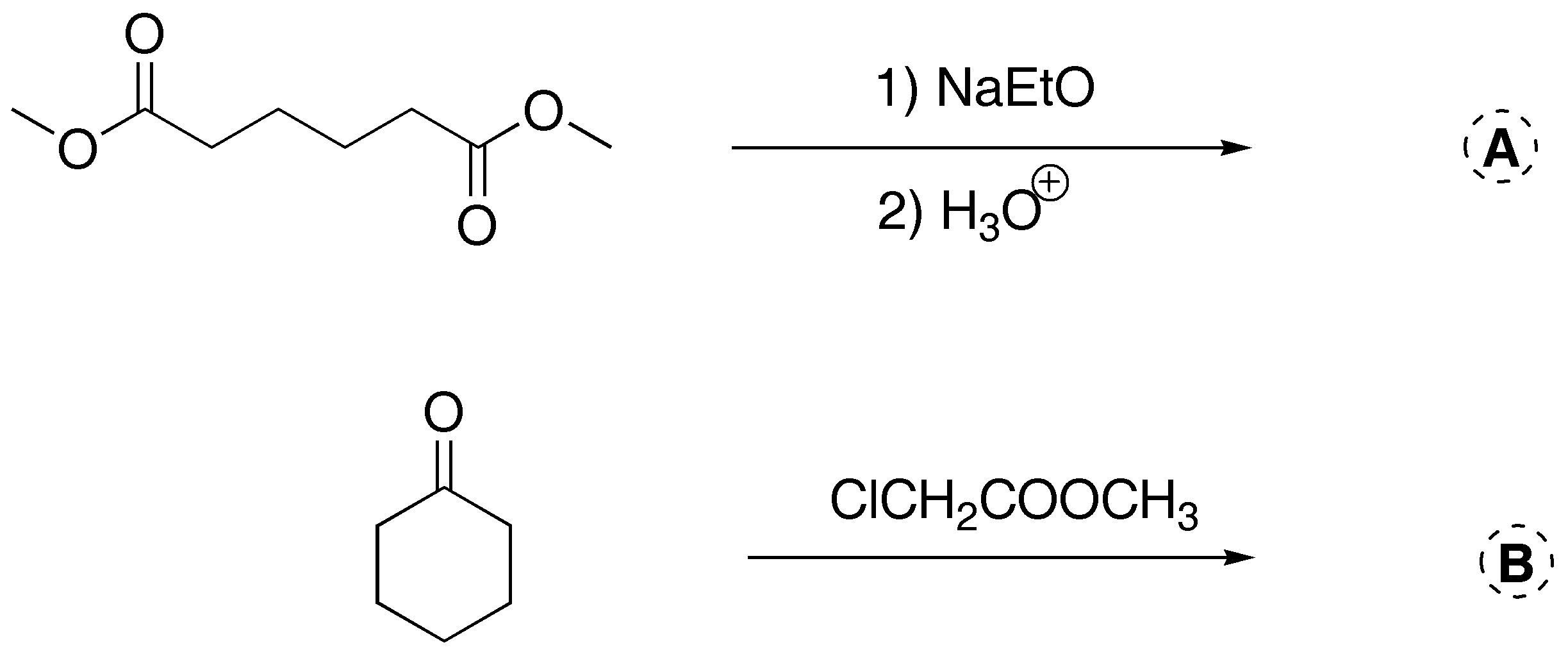### Problem 4)

Using cross aldol condensation reactions, what compounds would we need to prepare the following products?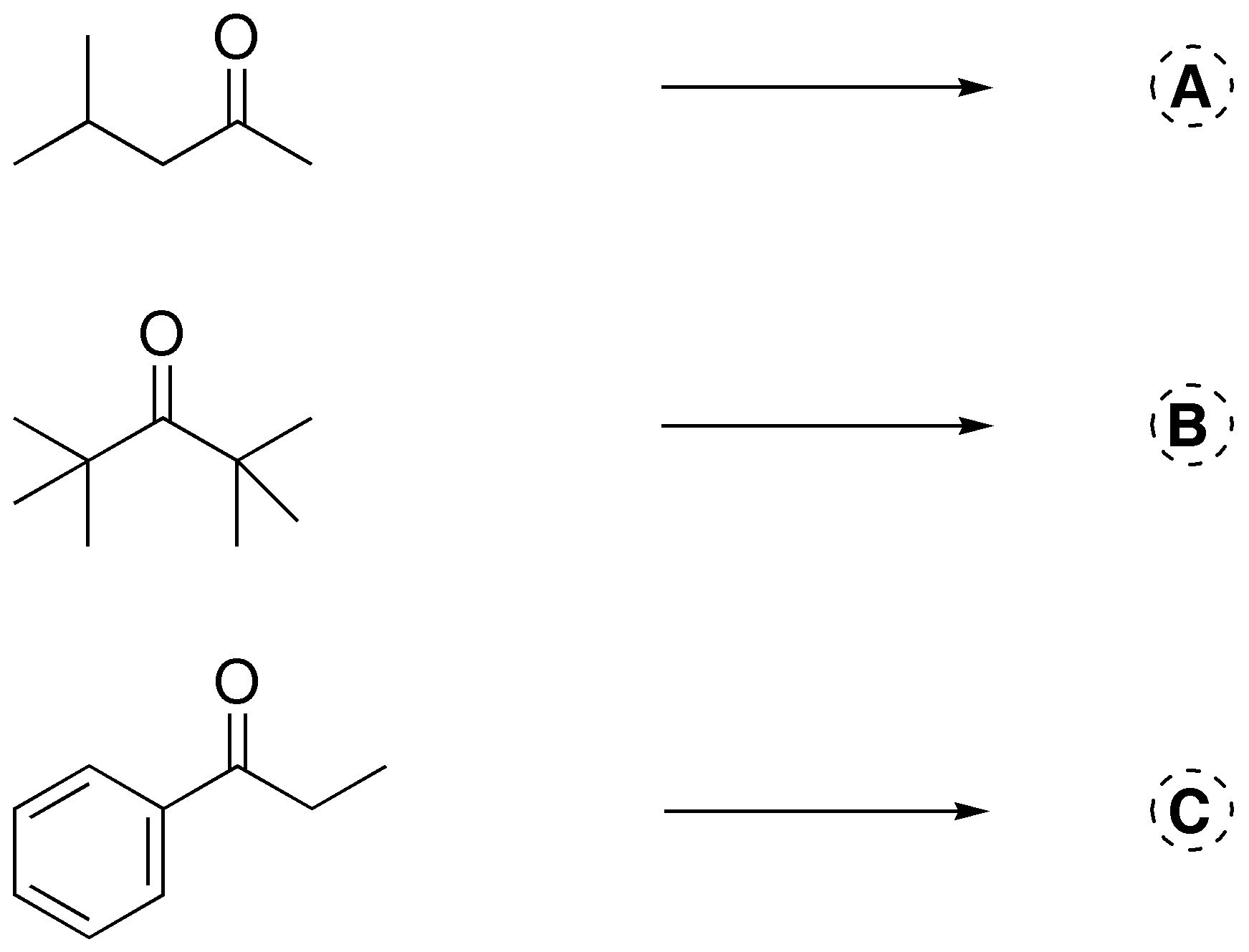### Problem 5)

Describe the starting compounds and reagents that could be used to prepare, by cross aldol condensation, the following products:

a) For the first reaction, acetone and benzaldehyde are required. First the acetone is treated with the base and then one mole of benzaldehyde is added, in this order, since if all the reagents are mixed, the Cannizzaro reaction would compete with the condensation reaction.

b) To obtain the product it is necessary to react formaldehyde with benzaldehyde in a basic medium, to give the condensation product, which is a keto-alcohol, which will present a strong tendency to dehydrate and form a conjugated double bond with the carbonyl group.

c) The product obtained is the result of the condensation between cyclohexanone and benzaldehyde.

d) It comes from the reaction between the carbanion obtained from acetone on formaldehyde.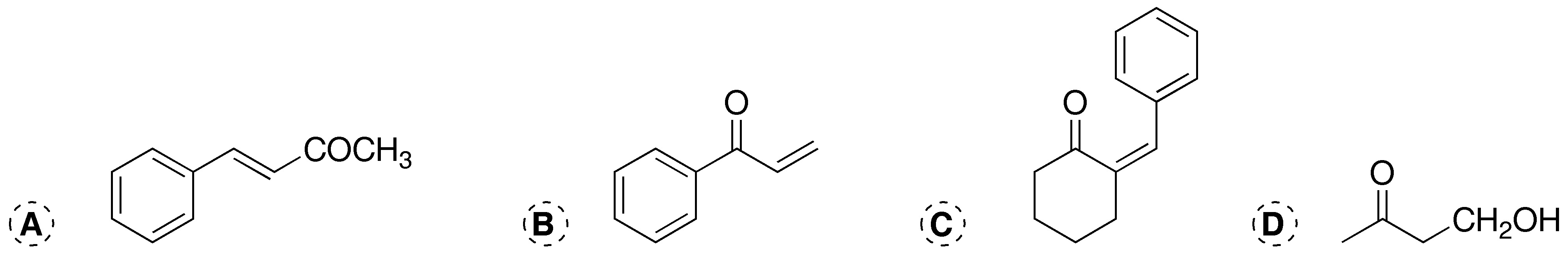### Problem 6)

Complete the following reactions:

All reactions are Michael-type additions where the acceptor is activated with an ethyl ester, nitrile, ketone and methyl ester group. Michael donors are carbanions of various nature and a primary amine.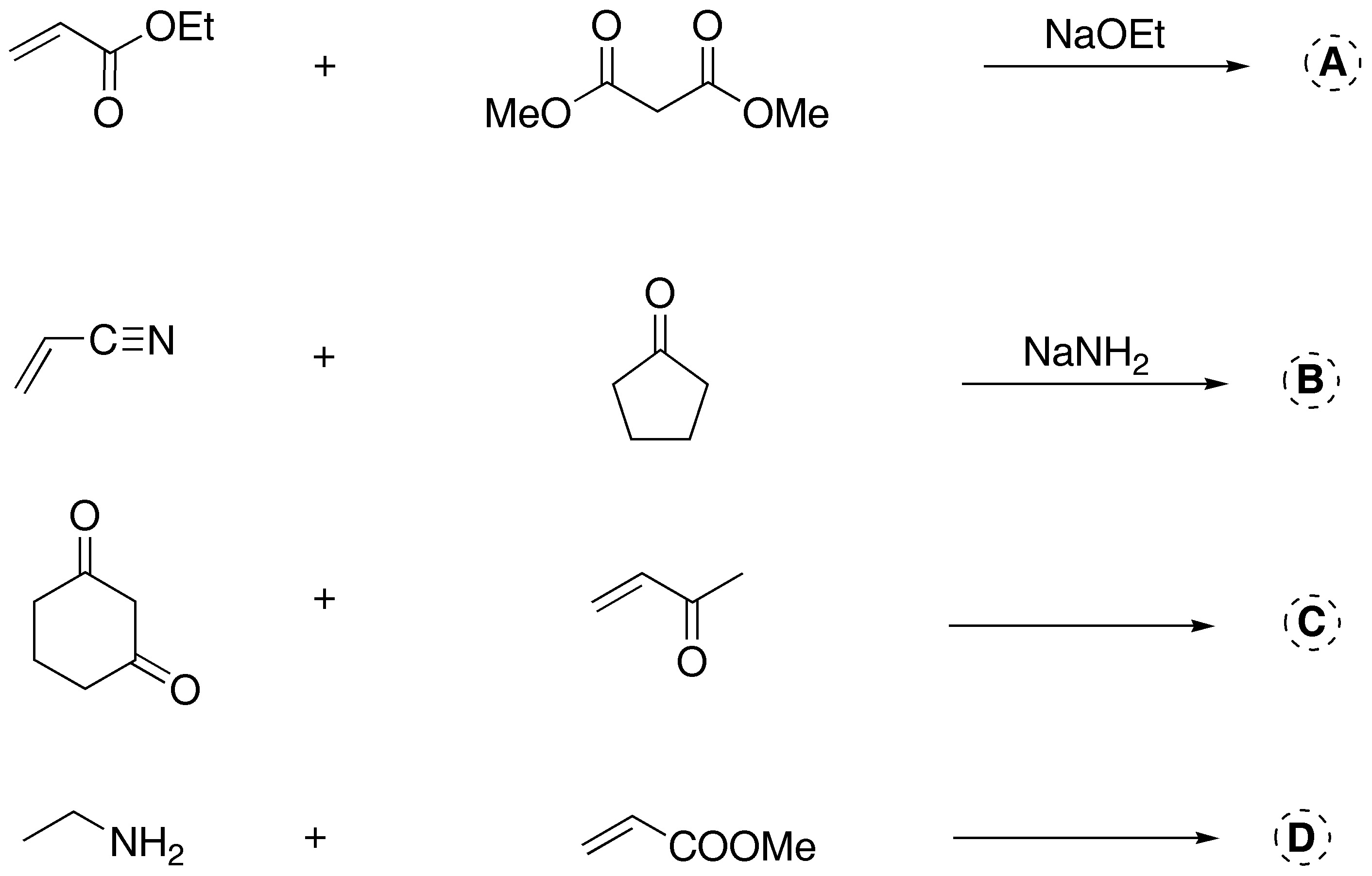### Problem 7)

Order the compounds with hydrogen-α according to their acidity (write in bold the α-hydrogen), Indicating what kind of base you would use to form the corresponding enolate (NaOH or else NaOEt).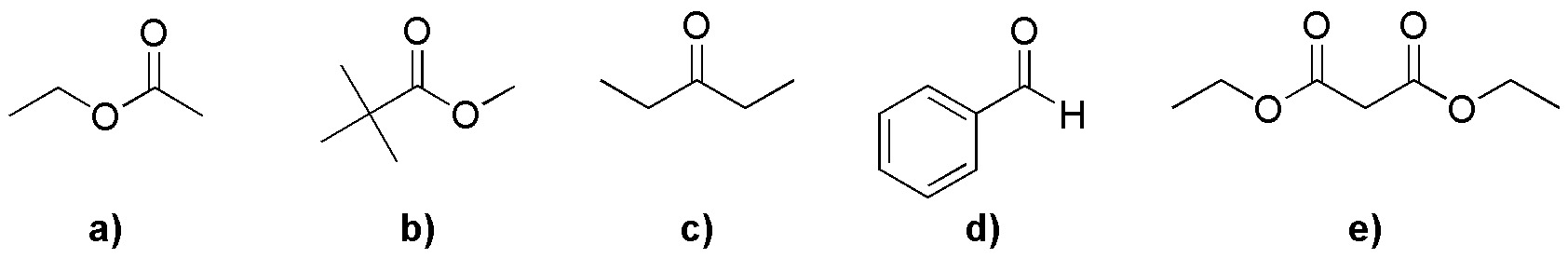### Problem 8)

Write the product/s obtained in the following Dieckmann cyclization: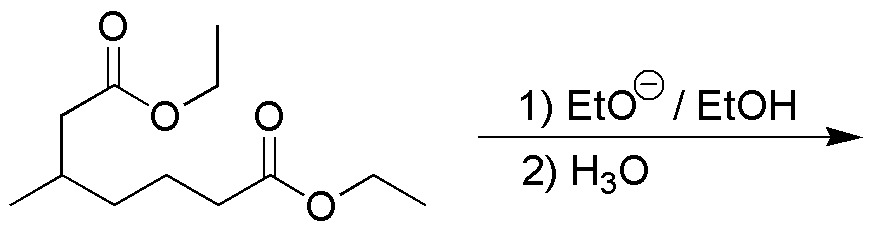### Problem 9)

Write the four aldoles obtained in the following cross aldol condensation: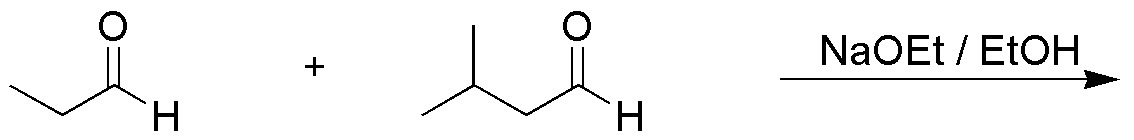### Problem 10)

Write the aldoles obtained in the following cross aldol condensations.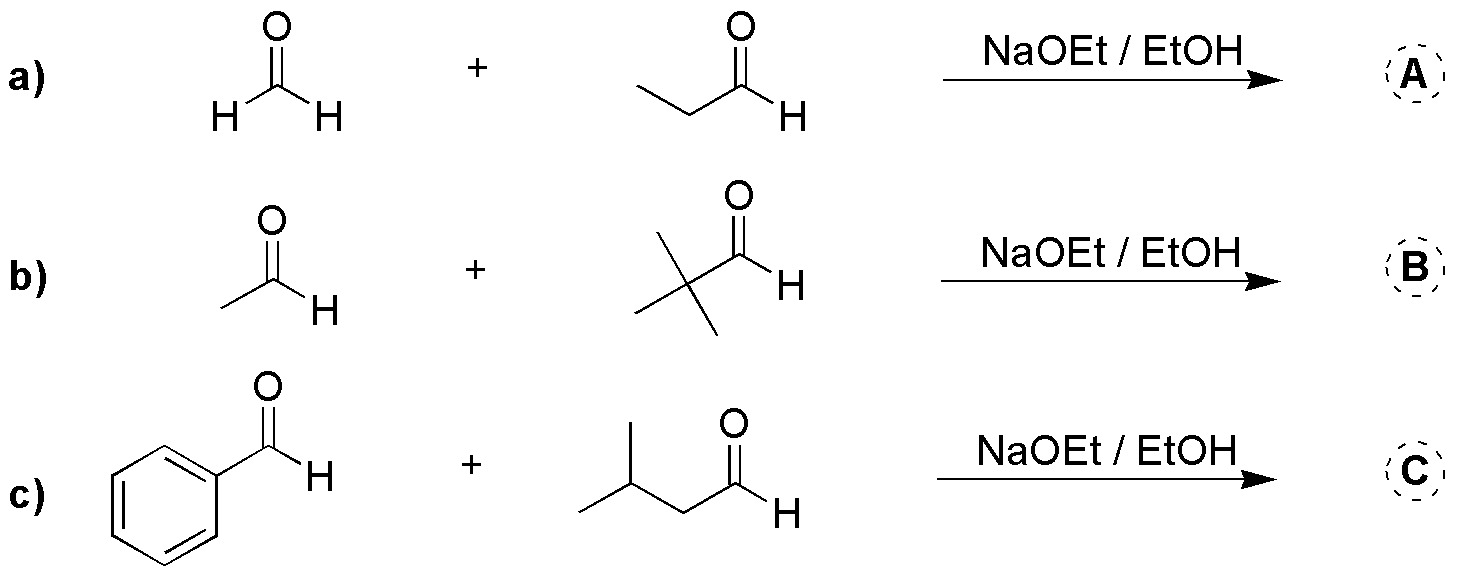### Problem 11)

Describe what major product is obtained in the following reaction: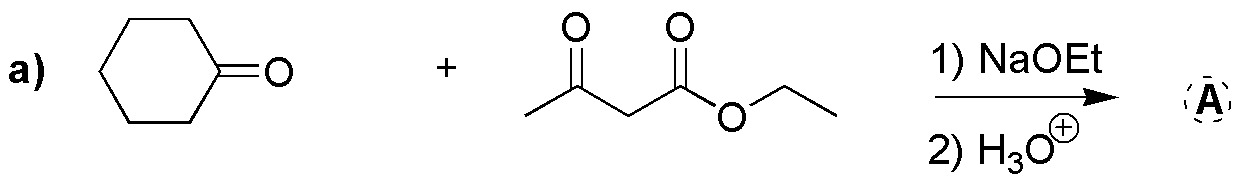### Problem 12)

Complete the following reactions (a-b).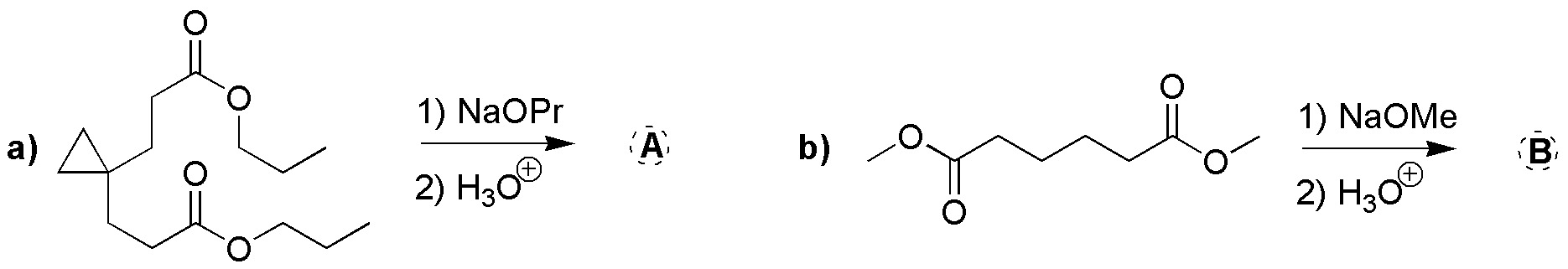### Problem 13)

Complete the following reactions (a-d).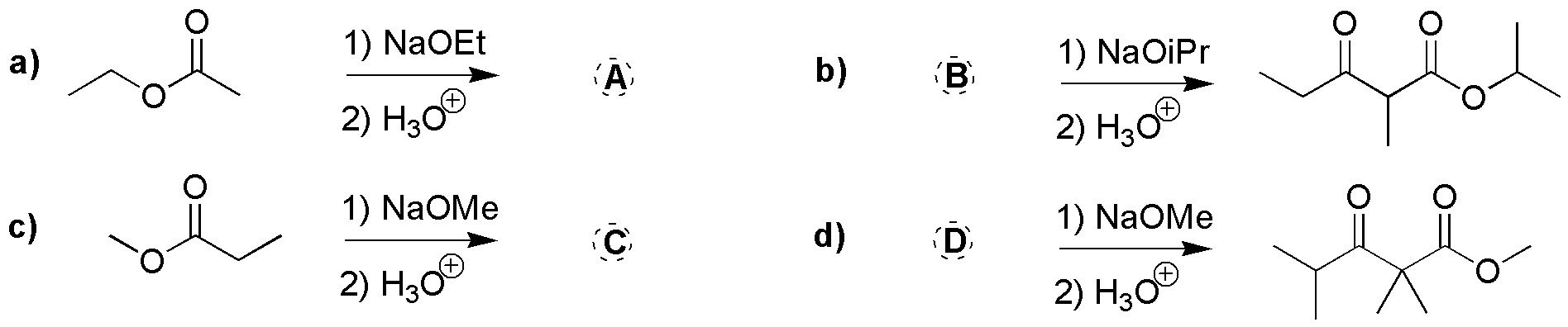### Problem 14)

Complete the following reaction, also indicating the intermediate that is formed.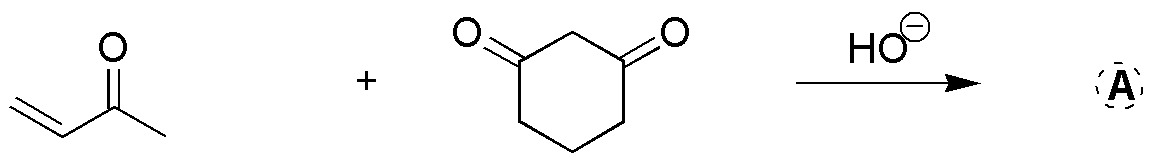### Problem 15)

Complete the following scheme giving the products for the following reactions.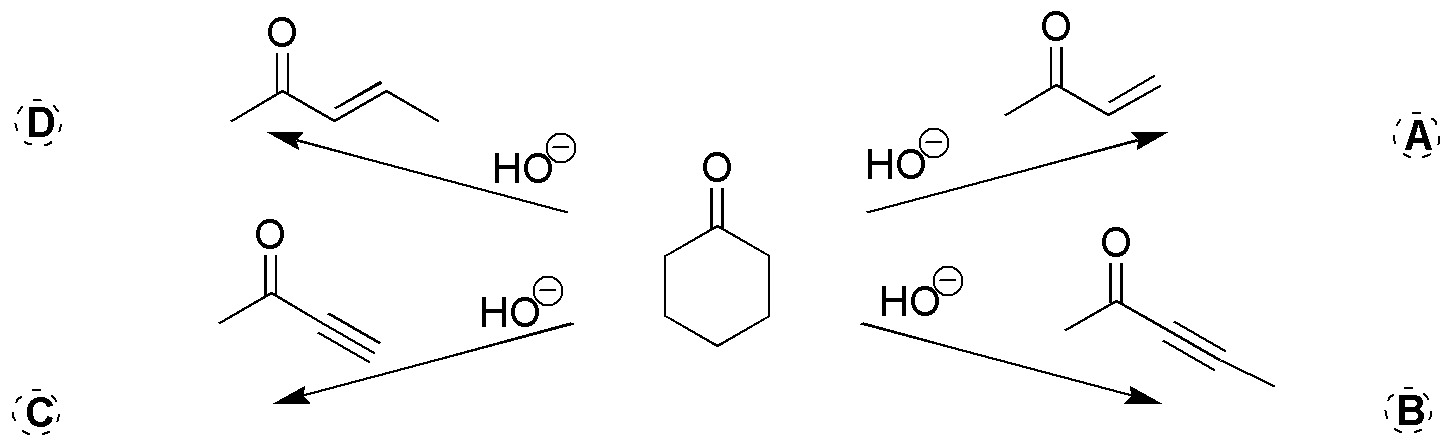### Problem 16)

Complete the following scheme giving the reactants for the following reactions: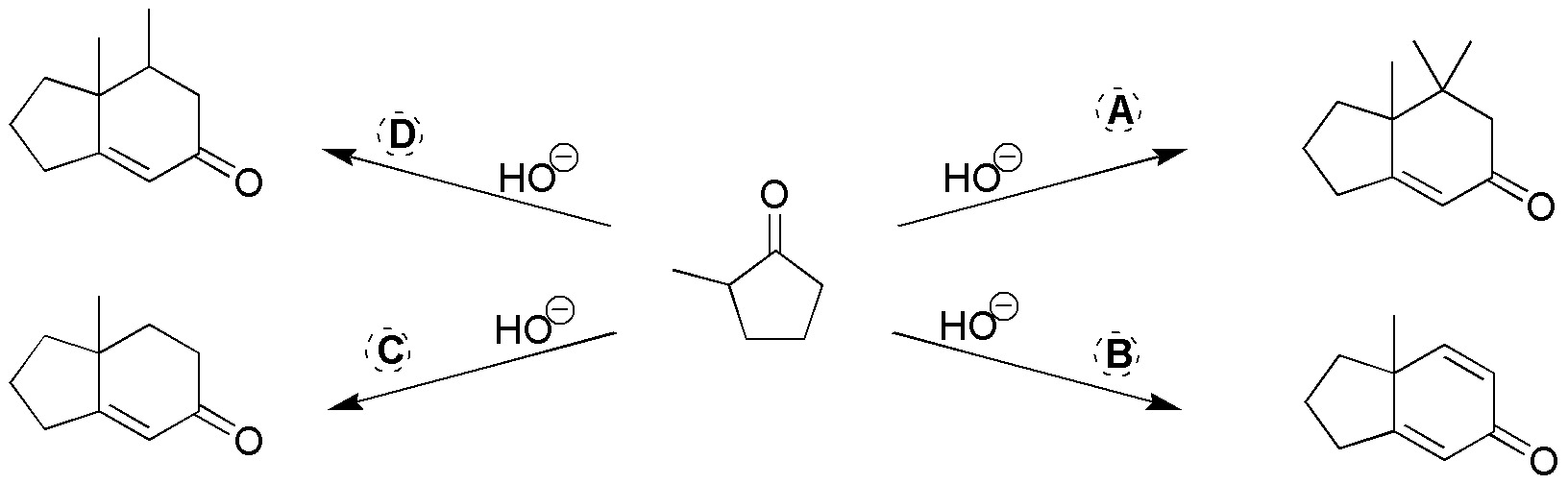### Problem 17)

Point out in boldface the α-hydrogens of the following compounds: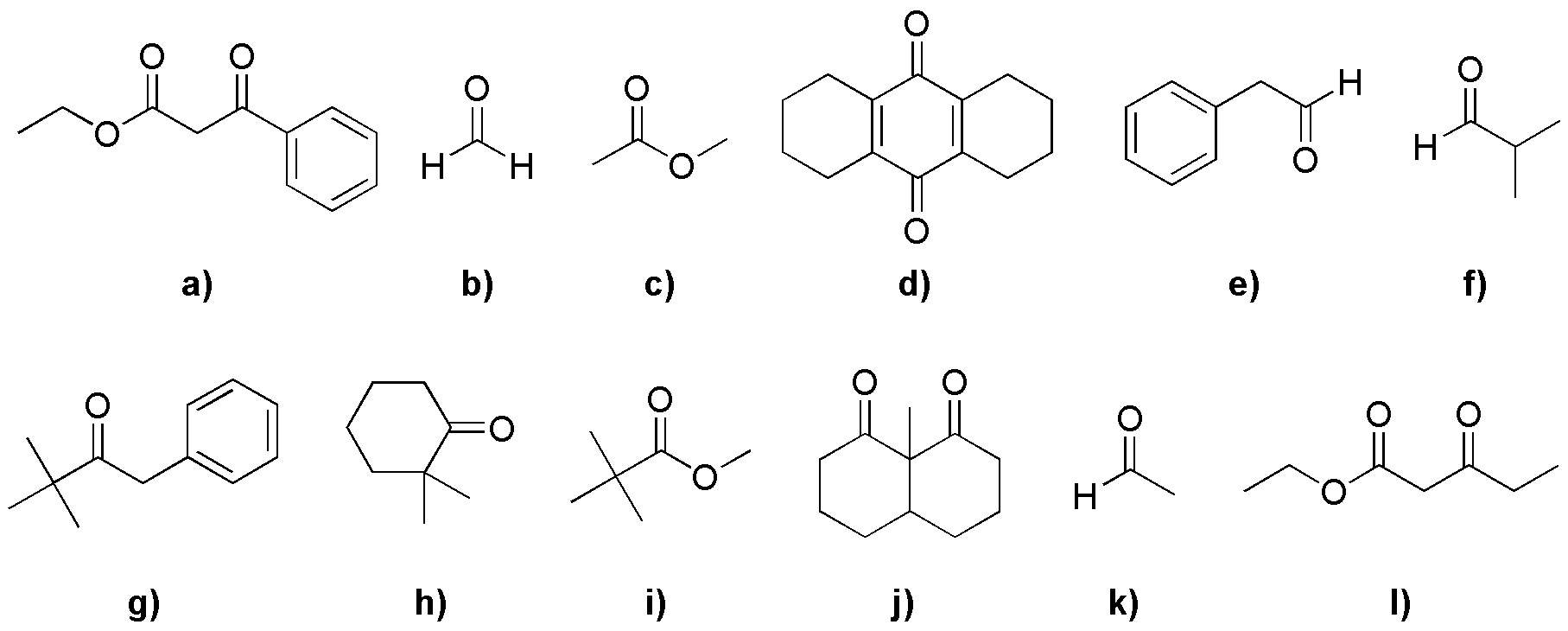### Problem 18)

Point out the most acidic hydrogens of the following compounds: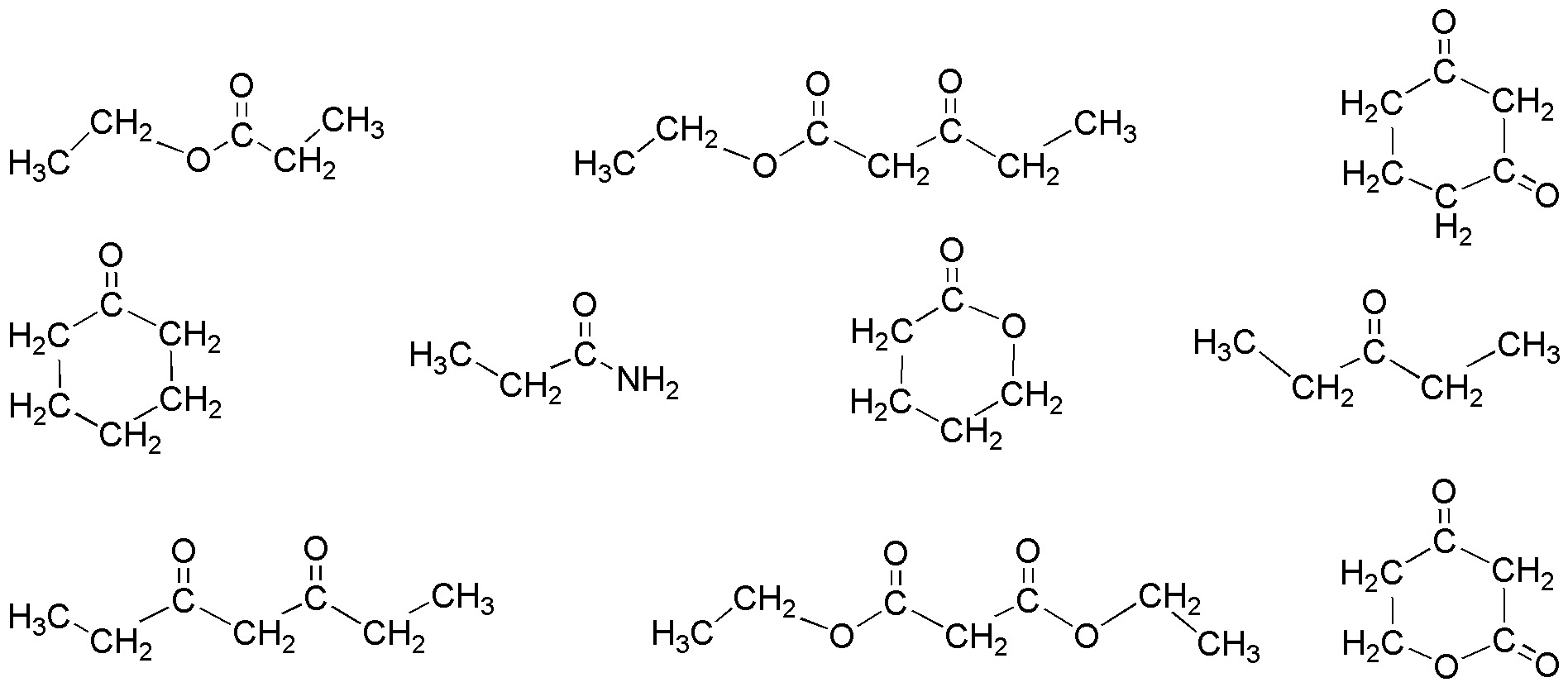### Problem 19)

Write the product/s obtained in the following reaction: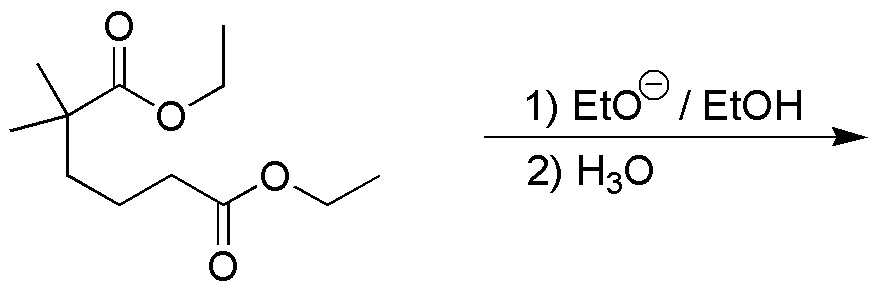### Problem 20)

Write the four aldoles obtained in the following reaction: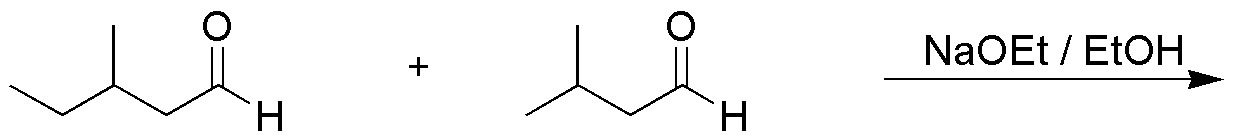### Problem 21)

Write the major product obtained in the following reaction: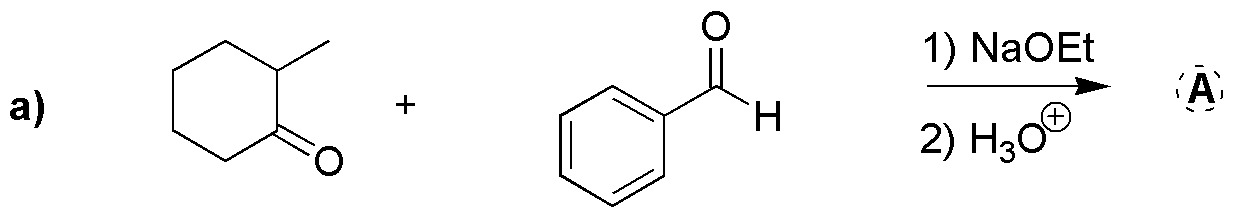### Problem 22)

Complete the following reactions (a-b):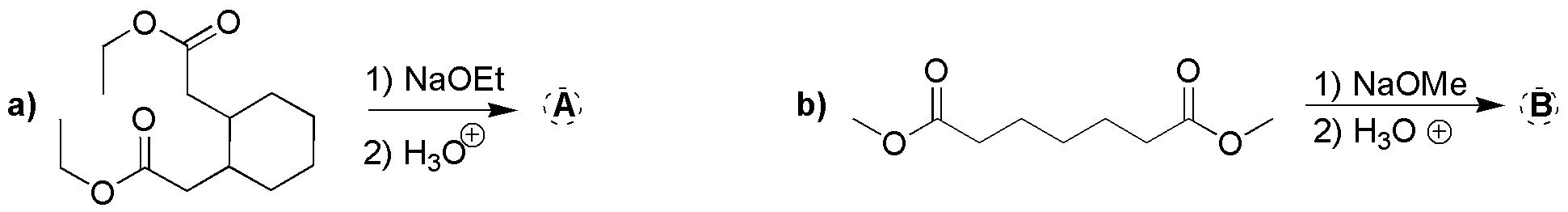### Problem 23)

Complete the following reactions (a-d):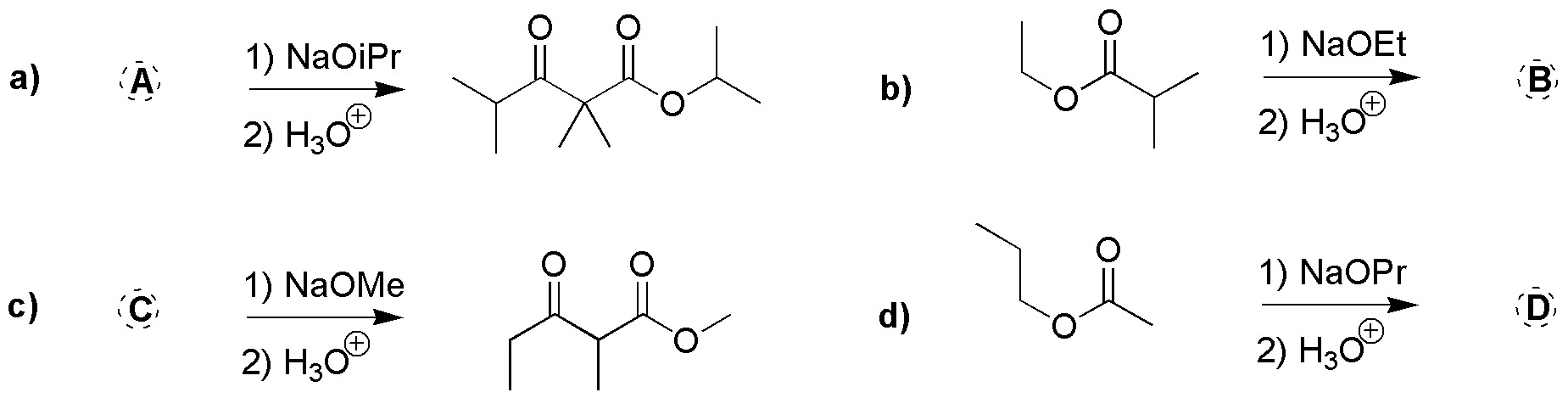### Problem 24)

Complete the following scheme by giving the reactants for the following reactions: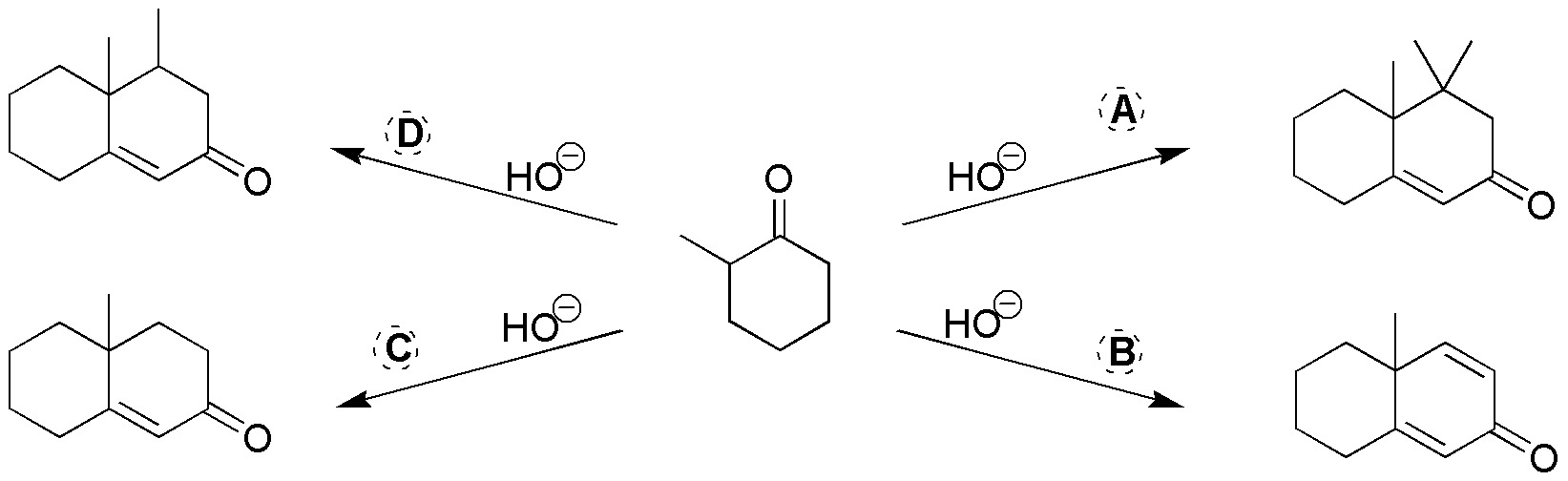### Problem 25)

Complete the following scheme by giving the products for the following reactions: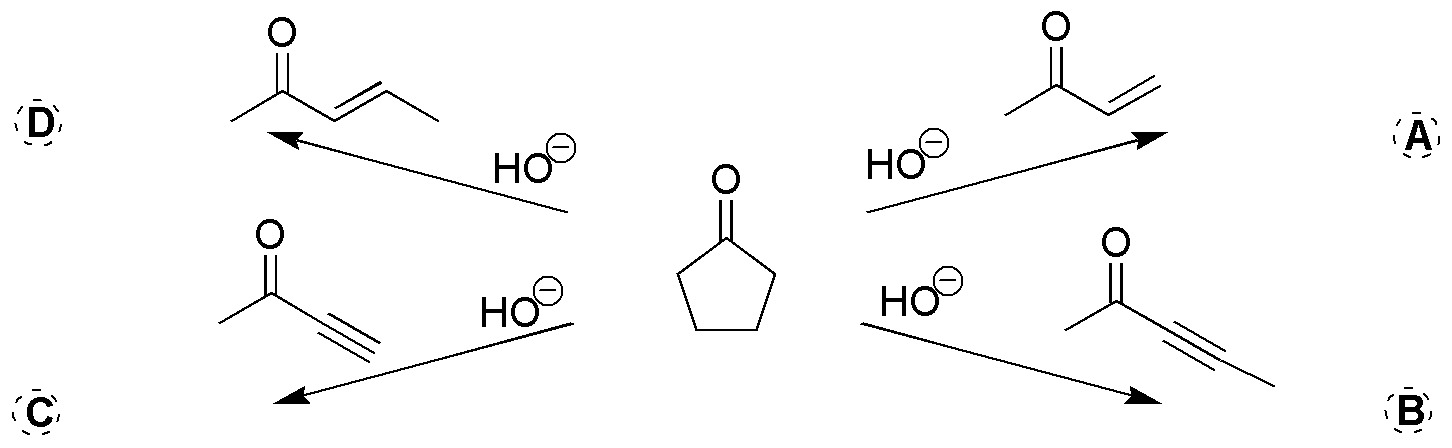### Problem 26)

Complete the following reaction, also indicating the intermediate that is formed: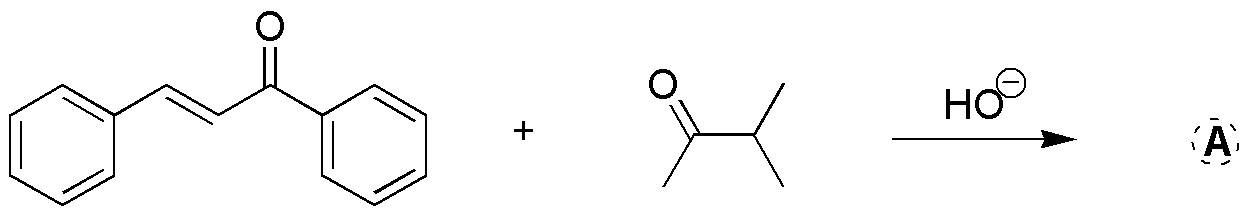### Problem 27)

Complete the following reactions and indicate the type to which each reaction belongs: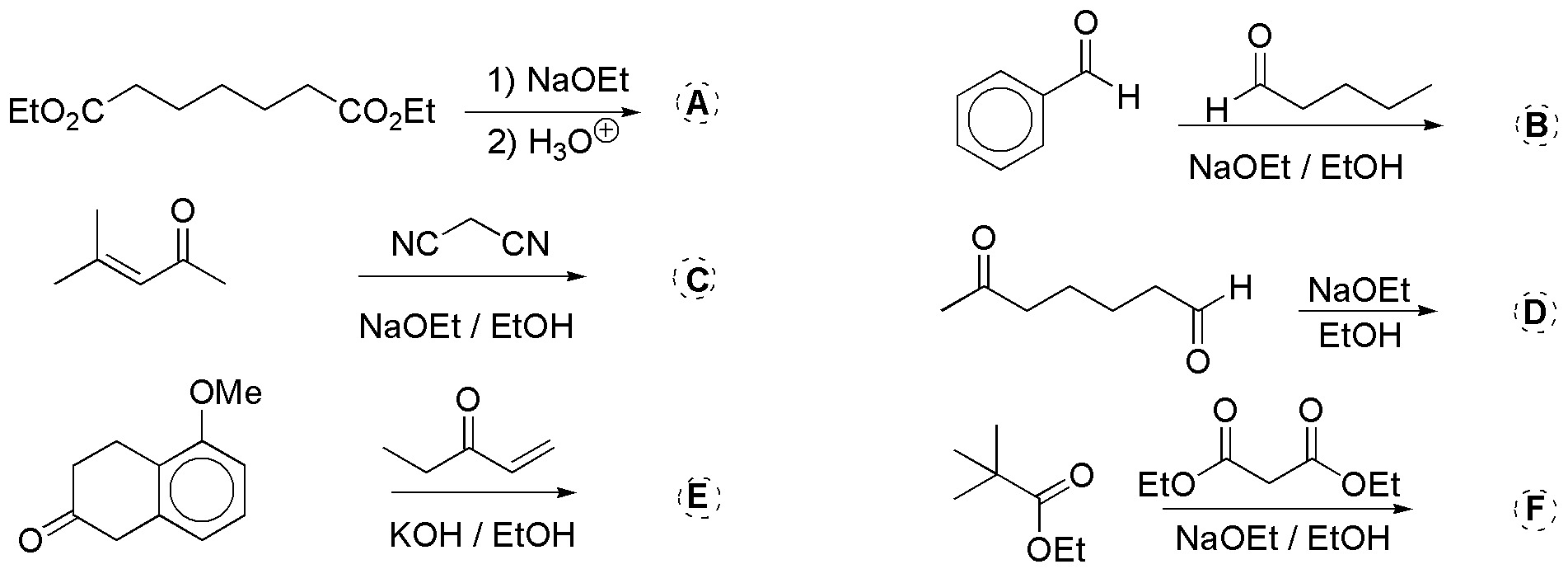### Problem 28)

Complete the following reactions (a-b).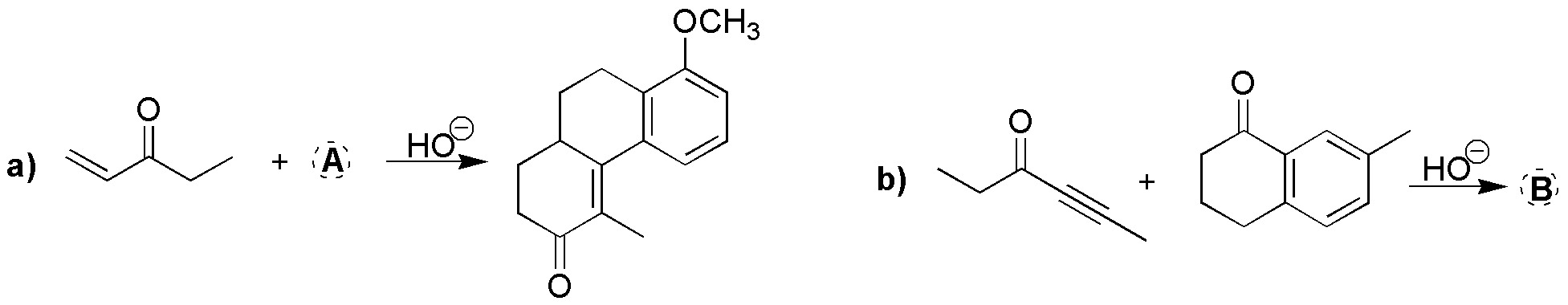Shares